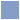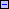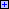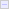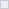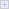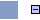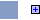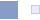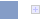• Maia Naftali
• Osvaldo Clua

# Introduction

• What has been done?
• Implement existing metrics in an accessibility evaluation tool.
• Web Accessibility Barrier Score
• Failure Rate
• Unified Web Evaluation Methodology Score
• The evaluation process is semi-automatic: includes a human filter.
• What for?
• Compare results from different sites.
• Analyze in a real scenario the difficulties of calculating metrics automatically.

# Difficulties found

• Major Difficulties
• Metric accuracy:
• Exact formulas with a variable input: how to achieve repeatable results when including human criteria.
• Human Filtering is useful, but requires extra work and depends on evaluator's knowledge, which is not ideal to implement in a large-scale scenario.
• Extra parameters:
• Calculating some parameters of the formulas that are not directly retrieved with the evaluation results can introduce an error. For example, the Failure Points.
• Threshold criteria and tool accuracy:
• Guideline checkpoints that are hard to test with an algorithm might add noise to metrics computing.
• Not all the checkpoints are tested in some evaluation tools.

# Ideas to work on for metric integration

• Metric categorization into levels:
• A possible categorization
• Basic: These metrics should only use the checkpoints that can be assessed automatically (with an algorithm). For example: Does the ALT tag has the IMG tag?.
• Semantic or extended: Metrics that use the entire set.
• Pragmatic: Metrics that measure the user experience.
• Motivation: automatic tools will be able to calculate the metrics defined as "basic", with a known error rate.

Limiting the scope in the metrics input will facilitate their programmatic implementation. Therefore, any evaluation tool could calculate metrics at a know level of accuracy.